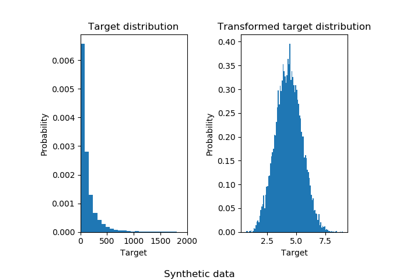# sklearn.preprocessing.quantile_transform¶

sklearn.preprocessing.quantile_transform(X, axis=0, n_quantiles=1000, output_distribution='uniform', ignore_implicit_zeros=False, subsample=100000, random_state=None, copy='warn')[source]

Transform features using quantiles information.

This method transforms the features to follow a uniform or a normal distribution. Therefore, for a given feature, this transformation tends to spread out the most frequent values. It also reduces the impact of (marginal) outliers: this is therefore a robust preprocessing scheme.

The transformation is applied on each feature independently. First an estimate of the cumulative distribution function of a feature is used to map the original values to a uniform distribution. The obtained values are then mapped to the desired output distribution using the associated quantile function. Features values of new/unseen data that fall below or above the fitted range will be mapped to the bounds of the output distribution. Note that this transform is non-linear. It may distort linear correlations between variables measured at the same scale but renders variables measured at different scales more directly comparable.

Read more in the User Guide.

Parameters
Xarray-like, sparse matrix

The data to transform.

axisint, (default=0)

Axis used to compute the means and standard deviations along. If 0, transform each feature, otherwise (if 1) transform each sample.

n_quantilesint, optional (default=1000 or n_samples)

Number of quantiles to be computed. It corresponds to the number of landmarks used to discretize the cumulative distribution function. If n_quantiles is larger than the number of samples, n_quantiles is set to the number of samples as a larger number of quantiles does not give a better approximation of the cumulative distribution function estimator.

output_distributionstr, optional (default=’uniform’)

Marginal distribution for the transformed data. The choices are ‘uniform’ (default) or ‘normal’.

ignore_implicit_zerosbool, optional (default=False)

Only applies to sparse matrices. If True, the sparse entries of the matrix are discarded to compute the quantile statistics. If False, these entries are treated as zeros.

subsampleint, optional (default=1e5)

Maximum number of samples used to estimate the quantiles for computational efficiency. Note that the subsampling procedure may differ for value-identical sparse and dense matrices.

random_stateint, RandomState instance or None, optional (default=None)

If int, random_state is the seed used by the random number generator; If RandomState instance, random_state is the random number generator; If None, the random number generator is the RandomState instance used by np.random. Note that this is used by subsampling and smoothing noise.

copyboolean, optional, (default=”warn”)

Set to False to perform inplace transformation and avoid a copy (if the input is already a numpy array). If True, a copy of X is transformed, leaving the original X unchanged

Deprecated since version 0.21: The default value of parameter copy will be changed from False to True in 0.23. The current default of False is being changed to make it more consistent with the default copy values of other functions in sklearn.preprocessing. Furthermore, the current default of False may have unexpected side effects by modifying the value of X inplace

Returns
Xtndarray or sparse matrix, shape (n_samples, n_features)

The transformed data.

QuantileTransformer

Performs quantile-based scaling using the Transformer API (e.g. as part of a preprocessing sklearn.pipeline.Pipeline).

power_transform

Maps data to a normal distribution using a power transformation.

scale

Performs standardization that is faster, but less robust to outliers.

robust_scale

Performs robust standardization that removes the influence of outliers but does not put outliers and inliers on the same scale.

Notes

NaNs are treated as missing values: disregarded in fit, and maintained in transform.

For a comparison of the different scalers, transformers, and normalizers, see examples/preprocessing/plot_all_scaling.py.

Examples

>>> import numpy as np
>>> from sklearn.preprocessing import quantile_transform
>>> rng = np.random.RandomState(0)
>>> X = np.sort(rng.normal(loc=0.5, scale=0.25, size=(25, 1)), axis=0)
>>> quantile_transform(X, n_quantiles=10, random_state=0, copy=True)
array([...])


## Examples using sklearn.preprocessing.quantile_transform¶